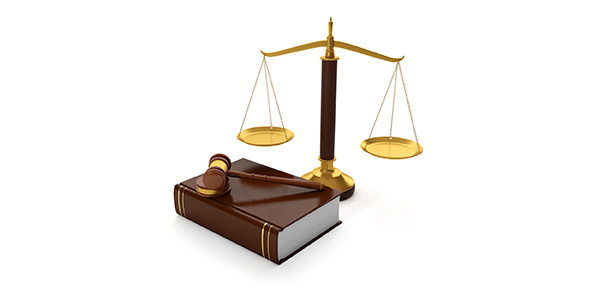# Boyles And Charles Law

12 Questions | Total Attempts: 665Settings• 1.
A balloon occupies a volume of 2.0 L at 40oC. How much volume will it occupy at 30oC?
• A.

1.5 L

• B.

1.94 L

• C.

1.6 L

• 2.
What is the final pressure in a balloon when the volume is changed from 1.5  L to a volume of 2.5L at constant temperature? (Initial pressure is 1.1 atm).
• A.

.66 atm

• B.

.66 kPa

• C.

4.125 atm

• 3.
Gas in a balloon occupies 3.3  L. What volume will it occupy if the pressure is changed from 100 kPa to 90  kPa (at constant temperature of 310 K (about room temperature).
• A.

29,700 L

• B.

2.97 L

• C.

3.6 L

• 4.
What is the final pressure in a vessel when the volume is changed from 1.3  L to a volume of 1.55 L at constant temperature? (Initial pressure is 1.5 atm).
• A.

1.25 L

• B.

1.65 L

• C.

1.49 L

• 5.
A balloon occupies 3.2 L at 37oC. How much will it occupy at 42oC?
• A.

3.15 L

• B.

3.25 L

• C.

312,480 L

• 6.
A gas occupies a balloon with a volume 2.0 L at 33oC. How much volume will it occupy at 37oC, if pressure remains constant.
• A.

1.97 L

• B.

186,000 L

• C.

2.06 L

• 7.
A gas expands from a volume 2.0 L at 36oC to a volume of 2.5 L, what is the final temperature, if the pressure is constant?
• A.

386 K ( which equals 113.25 Celsius)

• B.

247.2 K (which equals -25.8 C)

• C.

61.8 K (which equals -211.2 C)

• 8.
If the air inside a balloon is heated, the volume will:
• A.

Increase

• B.

Decrease

• C.

Increase a little, then decrease.

• 9.
According to the Kinetic Theory of Gases which is true
• A.

Gases are compressible because of the space between particles.

• B.

The distance between molecules in ideal gases is closer than in liquids or solids.

• C.

The gases are NOT fluid

• 10.
Which of the following describes the relationship between P and V?
• A.

Directly proportional

• B.

Inversely proportional

• C.

Unrelated

• 11.
Boyle's Law expresses the relationship between
• 12.
T1/P1 = T2/P2 is Charles's Law
• A.

True

• B.

False

Related TopicsBack to top